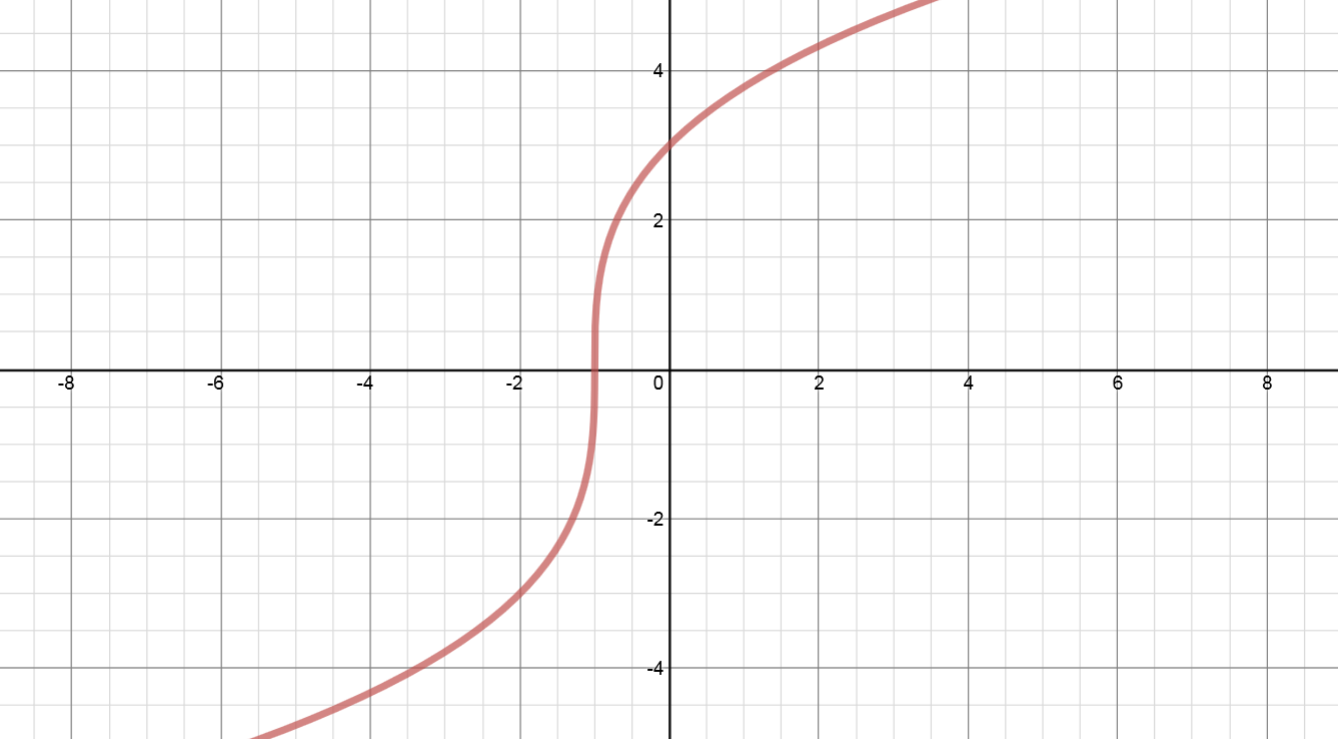# Transformations of functions: Horizontal translations#### You’re one step closer to a better grade.

Learn with less effort by getting unlimited access, progress tracking and more.Learn More

### Examples

#### Lessons

1. Sketch the following functions on the same set of coordinate axes:
$y = {\left( x \right)^2}$      VS.      $y = {\left( {x - 6} \right)^2}$      VS.      $y = {\left( {x + 5} \right)^2}$
2. Compared to the graph of $y = {x^2}$:
• the graph of $y = {\left( {x - 6} \right)^2}$ is translated "horizontally" ________ units to the ______________.
• the graph of $y = {\left( {x + 5} \right)^2}$ is translated "horizontally" ________ units to the ______________.
1. Horizontal Translations
Given the graph of $y = f\left( x \right)$ as shown, sketch:
1. $y = f\left( {x-8} \right)$
2. $y = f\left( {x+3} \right)$
3. In conclusion:
$\left( x \right) \to \left( {x-8} \right)$: shift __________ to the __________. All x coordinates $\Rightarrow$ ____________________
$\left( x \right) \to \left( {x+3} \right)$: shift __________ to the __________. All x coordinates $\Rightarrow$ ____________________## Become a Member to Get More!

• #### Easily See Your ProgressWe track the progress you've made on a topic so you know what you've done. From the course view you can easily see what topics have what and the progress you've made on them. Fill the rings to completely master that section or mouse over the icon to see more details.

• #### Make Use of Our Learning Aids###### Practice Accuracy

See how well your practice sessions are going over time.

Stay on track with our daily recommendations.

• #### Earn Achievements as You LearnMake the most of your time as you use StudyPug to help you achieve your goals. Earn fun little badges the more you watch, practice, and use our service.

• #### Create and Customize Your AvatarPlay with our fun little avatar builder to create and customize your own avatar on StudyPug. Choose your face, eye colour, hair colour and style, and background. Unlock more options the more you use StudyPug.

###### Topic Basics
Horizontal translations refer to movements of a graph of a function horizontally along the x-axis by changing the x values. So, if y = f(x), then y = (x –h) results in a horizontal shift. If h > 0, then the graph shifts h units to the right; while If h < 0, then the graph shifts h units to the left.
Compared to $y=f(x)$:
$y=f(x-8)$: shift 8 units to the right
$y=f(x+3)$: shift 3 units to the left